#Calculus Worksheets

## Differentiation Applications Worksheets

Here is a graphic preview for all of the Differentiation Applications for Calculus Worksheets. You can select different variables to customize these Differentiation Applications for Calculus Worksheets for your needs. The Differentiation Applications for Calculus Worksheets are randomly created and will never repeat so you have an endless supply of quality Differentiation Applications for Calculus Worksheets to use in the classroom or at home. We have rate of change, graphing, graph properties, differentials, optimization, Newton's Method, and related rates worksheets.

Our Differentiation Applications for Calculus Worksheets are free to download, easy to use, and very flexible.

These Differentiation Applications for Calculus Worksheets are a good resource for students in high school.

Click here for a Detailed Description of all the Differentiation Applications for Calculus Worksheets.

## Quick Link for All Differentiation Applications for Calculus Worksheets

Click the image to be taken to that Differentiation Applications for Calculus Worksheets.

##### Formulas for Rates of Change Worksheets##### Evaluate a Derivative at a Point Worksheets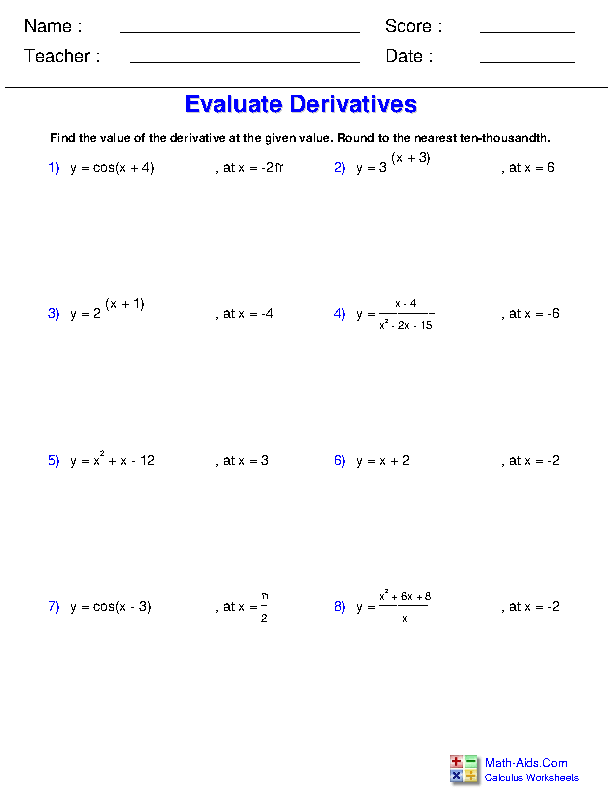##### Evaluate Slope at a Point Worksheets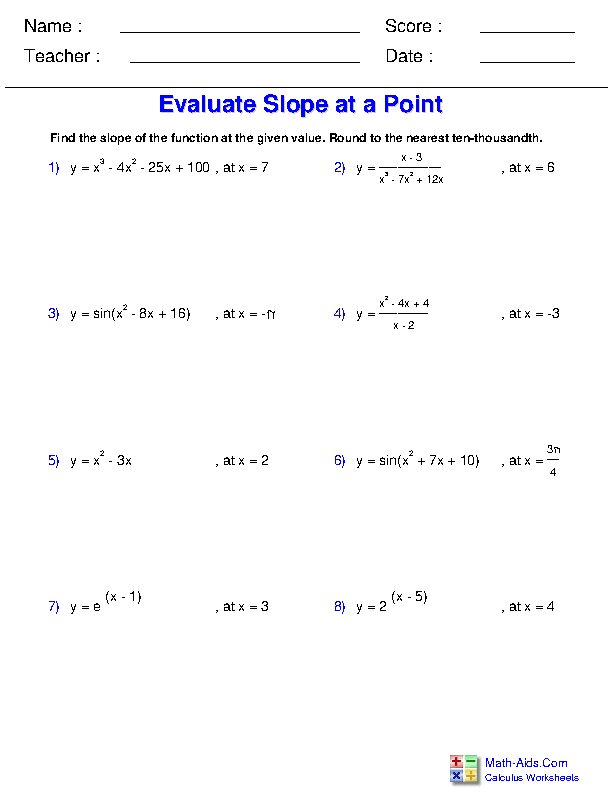##### Finding Points with Horizontal Tangents Worksheets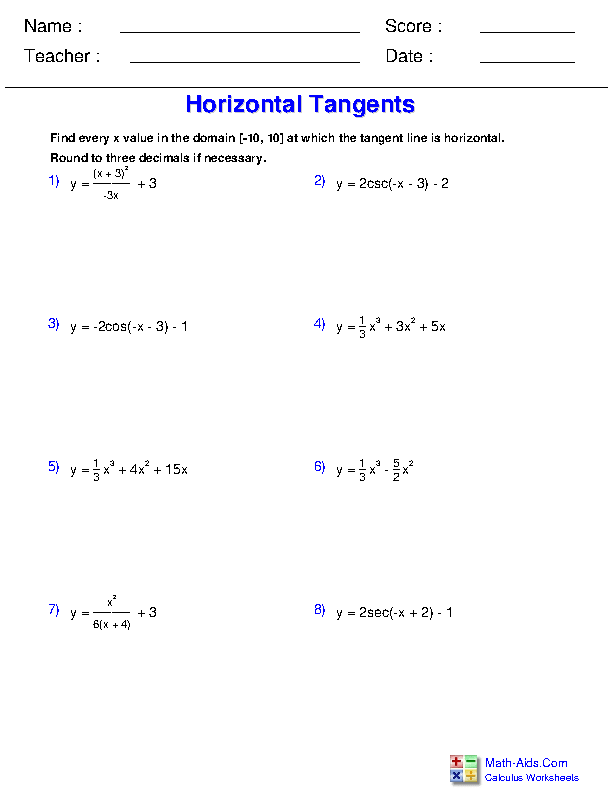##### Horizontal Tangents on Graphs Worksheets##### Identify Absolute Extrema Worksheets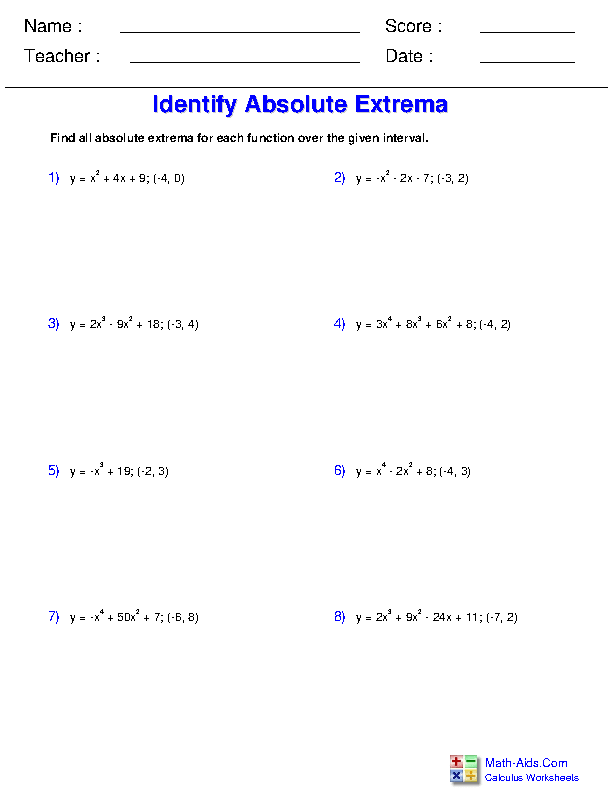##### Identify Relative Extrema Worksheets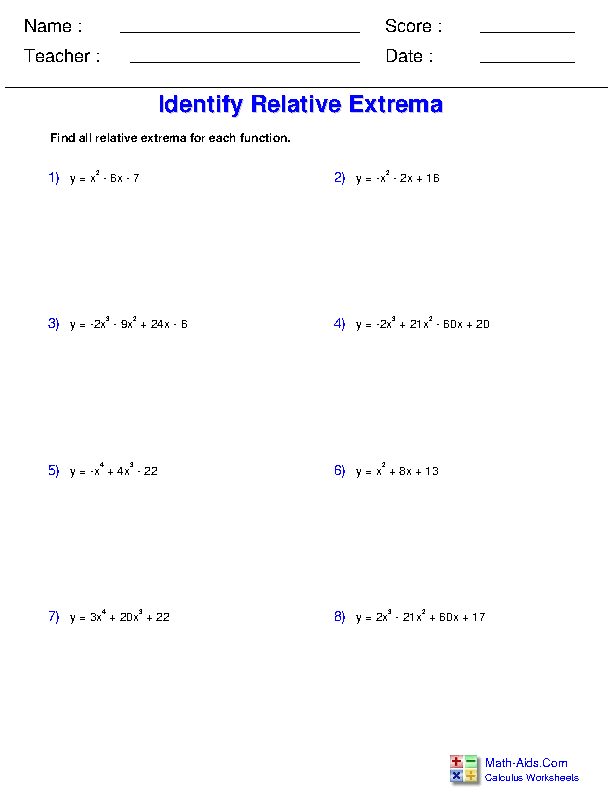##### Graph Derivative of a Function Worksheets##### Graphing from Function Properties Worksheets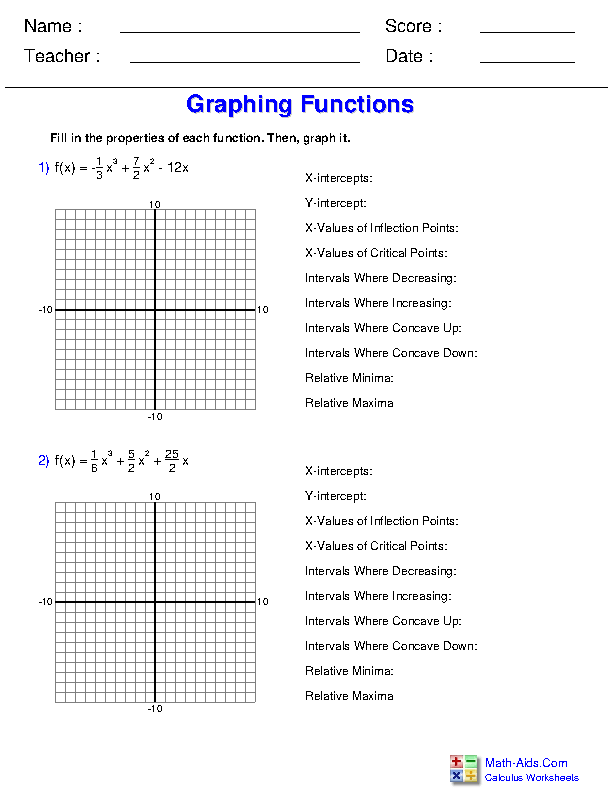##### Understanding Motion Worksheets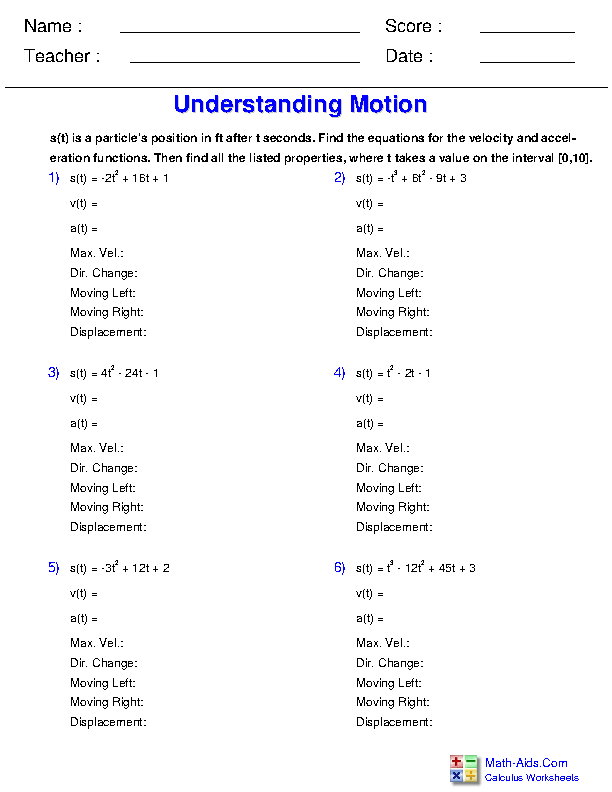##### Understanding Critical Points Worksheets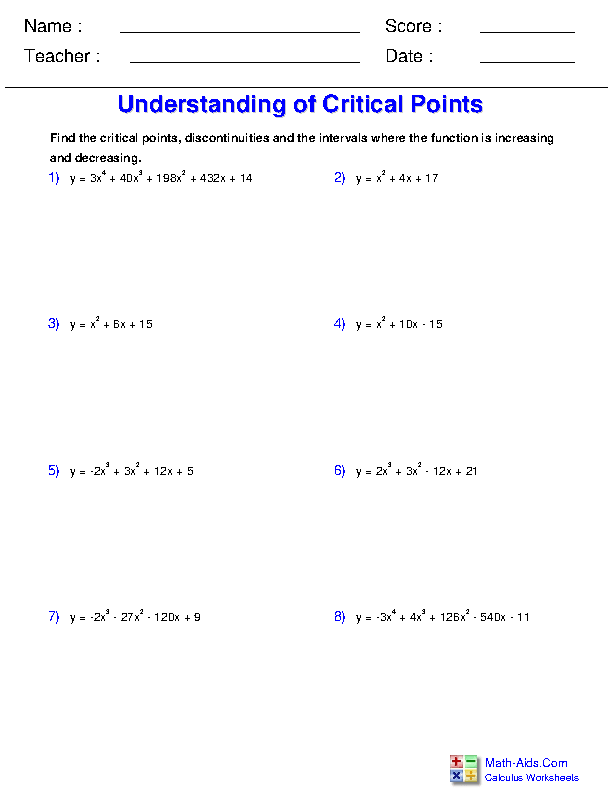##### Understanding Concavity Worksheets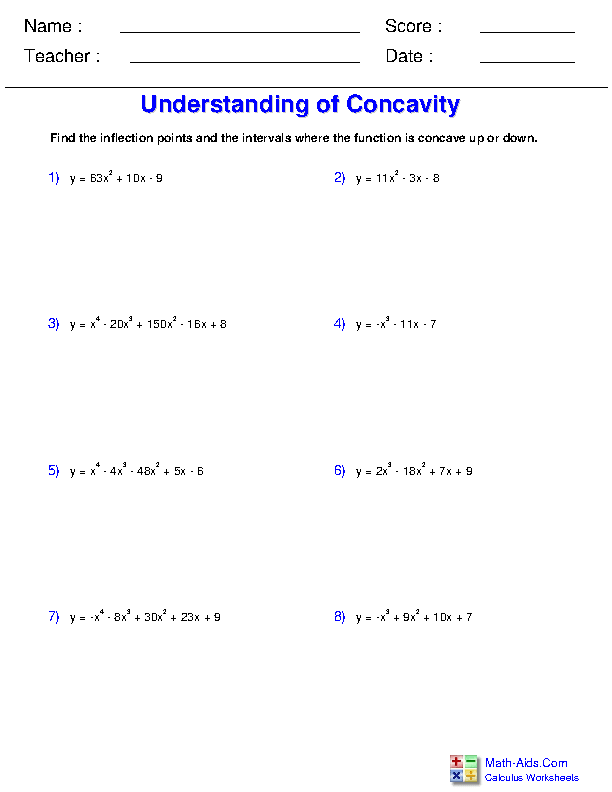##### L'Hopital's Rule Worksheets##### Differentials Worksheets##### Graphing Differentials Worksheets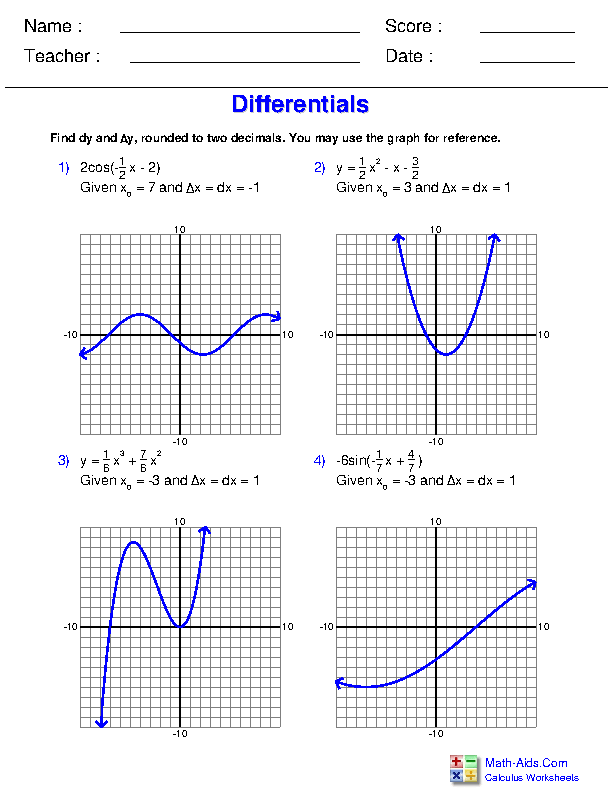##### Find the Tangent Line Worksheets##### Find the Normal Line Worksheets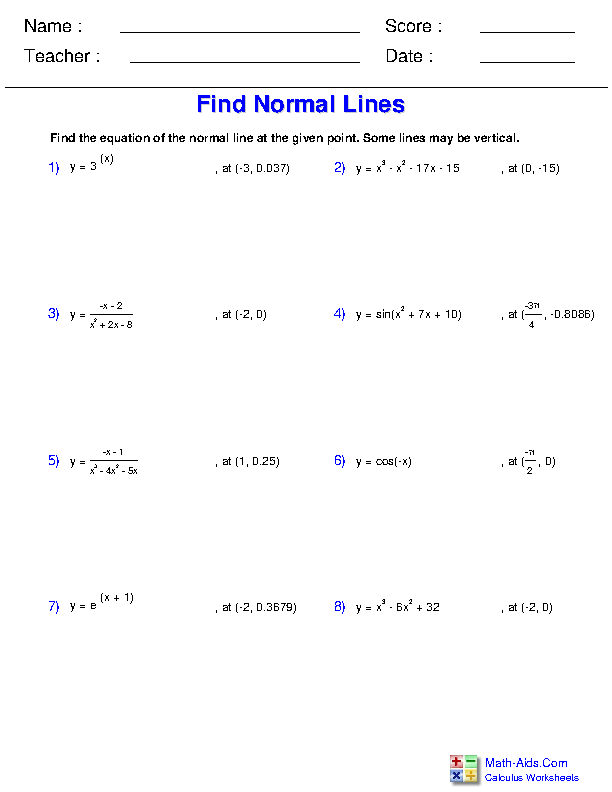##### Limits From the Definition of the Derivative Worksheets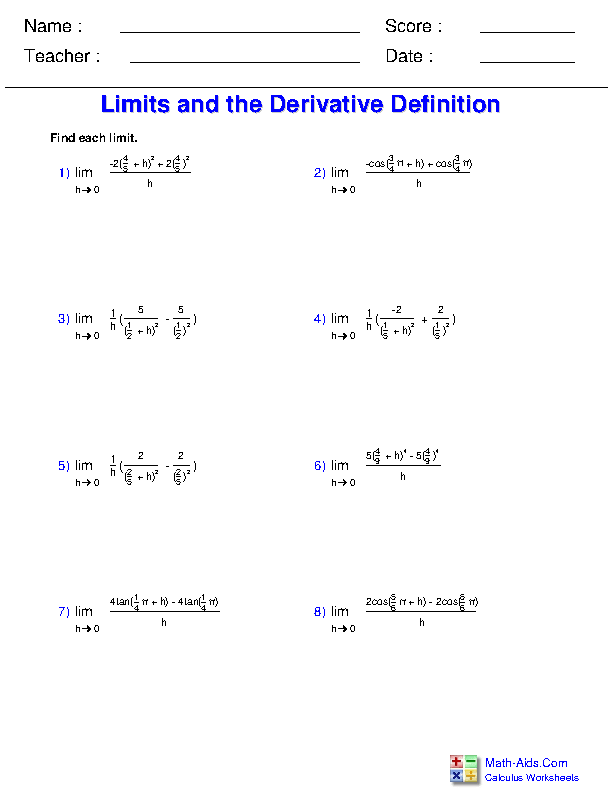##### Linear Approximation Worksheets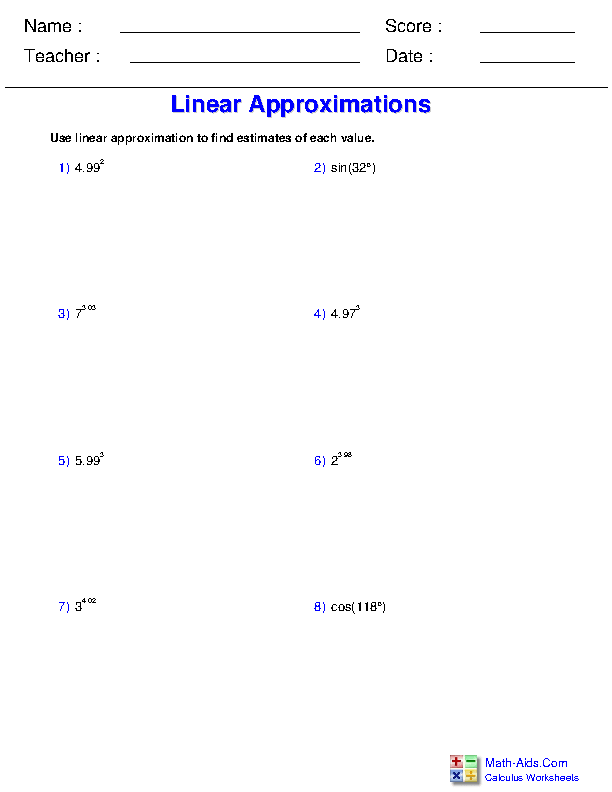##### Newton's Method Worksheets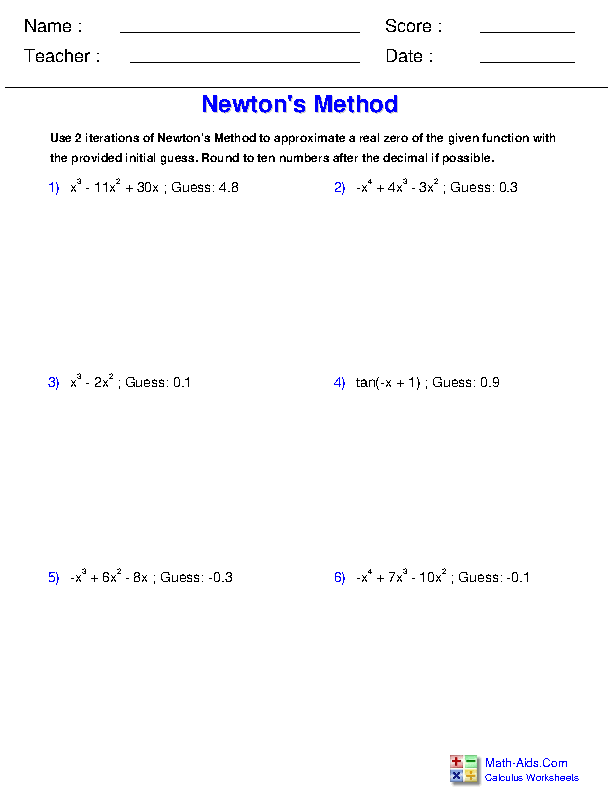##### Newton's Method with Root Functions Worksheets##### Optimization Worksheets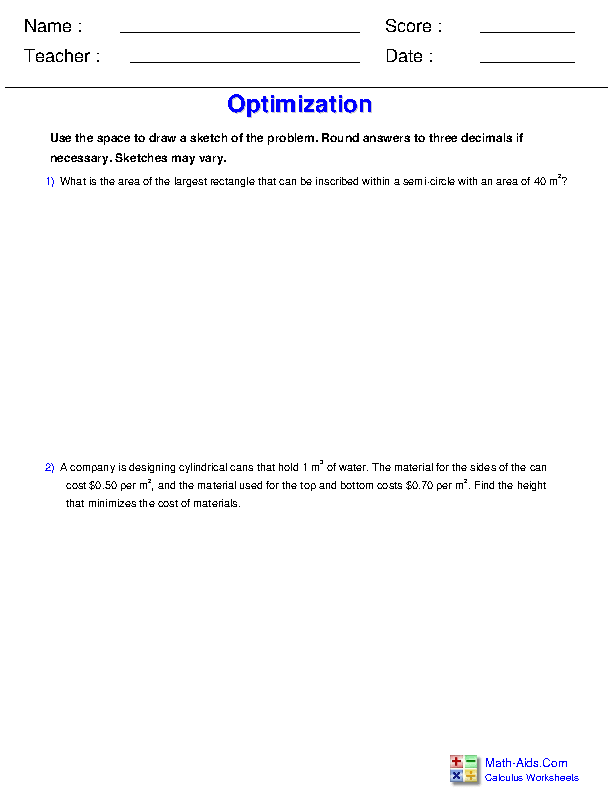##### Related Rates Worksheets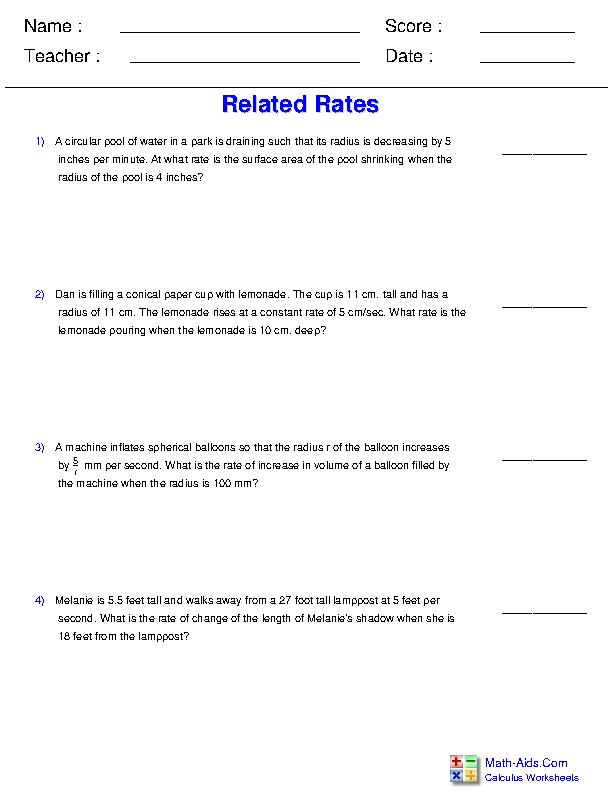Recommended Videos

## Detailed Description for All Differentiation Applications for Calculus Worksheets

Formulas for Rates of Change Worksheets
This Calculus - Differentiation Applications Worksheet will produce problems that deal with finding the formulas for the rates of change in functions.

Evaluate a Derivative at a Point Worksheets
This Calculus - Differentiation Applications Worksheet will produce problems that ask students to evaluate a derivative at a given point.

Evaluate Slope at a Point Worksheets
This Calculus - Differentiation Applications Worksheet will produce problems that ask students to evaluate the slope of a function at a given point.

Finding Points with Horizontal Tangents Worksheets
This Calculus - Differentiation Applications Worksheet will produce problems that deal with finding points in equations that yield horizontal tangents.

Horizontal Tangents on Graphs Worksheets
This Calculus - Differentiation Applications Worksheet will produce problems that deal with finding points in equations that yield horizontal tangents using graphs.

Identify Absolute Extrema Worksheets
This Calculus - Differentiation Applications Worksheet will produce problems that ask students to identify the absolute extrema of a function.

Identify Relative Extrema Worksheets
This Calculus - Differentiation Applications Worksheet will produce problems that ask students to identify the relative extrema of a function.

Graph Derivative of a Function Worksheets
This Calculus - Differentiation Applications Worksheet will produce problems that involve drawing a graph of the derivative of a function.

Graphing from Function Properties Worksheets
This Calculus - Differentiation Applications Worksheet will produce problems that deal with graphing functions based on their properties, such as intercepts and concavity.

Understanding Motion Worksheets
This Calculus - Differentiation Applications Worksheet will produce problems that involve understanding motion through manipulating position, velocity and acceleration functions.

Understanding Critical Points Worksheets
This Calculus - Differentiation Applications Worksheet will produce problems that involve understanding critical points.

Understanding Concavity Worksheets
This Calculus - Differentiation Applications Worksheet will produce problems that involve understanding concavity.

L'Hopital's Rule Worksheets
This Calculus - Differentiation Applications Worksheet will produce problems that ask students to use L'Hopital's Rule to solve limit problems.

Differentials Worksheets
This Calculus - Differentiation Applications Worksheet will produce problems that deal with finding differentials of equations.

Graphing Differentials Worksheets
This Calculus - Differentiation Applications Worksheet will produce problems that involve graphing differentials of equations.

Find the Tangent Line Worksheets
This Calculus - Differentiation Applications Worksheet will produce problems that ask students to find the tangent line of a function at a given point.

Find the Normal Line Worksheets
This Calculus - Differentiation Applications Worksheet will produce problems that ask students to find the normal line of a function at a given point.

Limits From the Definition of the Derivative Worksheets
This Calculus - Differentiation Applications Worksheet will produce problems that ask students to solve problems with limits that are in the form of the definition of the derivative.

Linear Approximation Worksheets
This Calculus - Differentiation Applications Worksheet will produce problems that ask students to use linear approximation to find values.

Newton's Method Worksheets
This Calculus - Differentiation Applications Worksheet will produce problems that involve using Newton's Method to find the roots of a given function.

Newton's Method with Root Functions Worksheets
This Calculus - Differentiation Applications Worksheet will produce problems that involve using Newton's Method with root functions to find square roots, cube roots and more.

Optimization Worksheets
This Calculus - Differentiation Applications Worksheet will produce word problems that deal with the optimization of resources in scenarios.

Related Rates Worksheets
This Calculus - Differentiation Applications Worksheet will produce word problems that deal with using related rates.